output.to from Sideway

Draft for Information Only

# Content

```  Law of Conservation   Continuity Equation, 1D   Euler's Equation of Inviscid Motion, 1D   Energy Equation, 1D ```

## Law of Conservation

Based on linear acoustic, assuming the cross-section area equals to A and no mass is entering or leaving the system due to the acoustic disturbance, the wave propagation can be represented by following figure: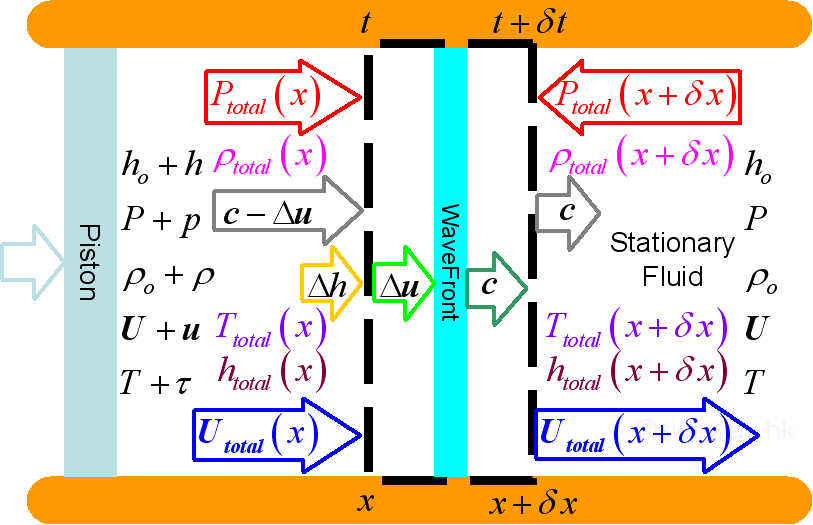where, at ambient environment and at acoustic disturbance state: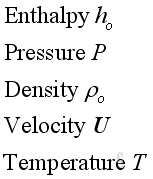and,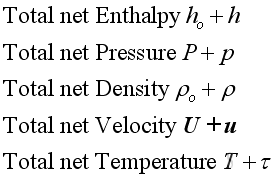where, properties of acoustic disturbance: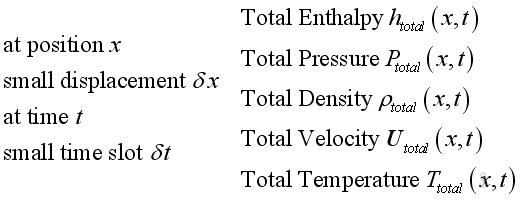where, properties at wavefront: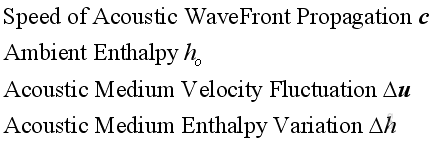## Continuity Equation, 1D

For a control volume, from the principle of conservation of mass, the instantaneous rate of change of mass in a control volume equals to the net mass flux flow into or out of the control volume, therefore: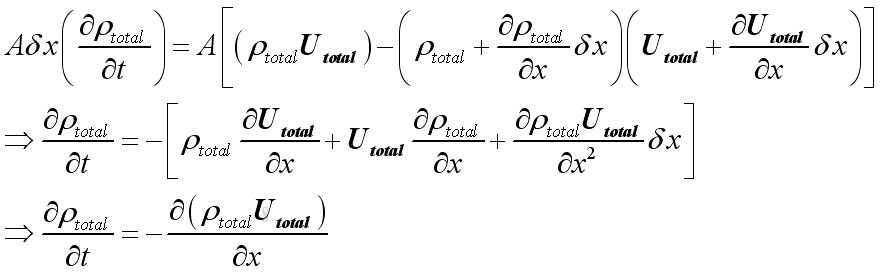The relationship between density and velocity is defined

## Euler's Equation of Inviscid Motion, 1D

For a control volume, from principle of momentum conservation, the instantaneous rate of change of net momentum of a control volume equals to the net applied force and the net momentum change due to the momentum flux flow into or out of the control volume. The  applied force in this case is pressure only and no other forces, no gravity, no viscous force etc., then: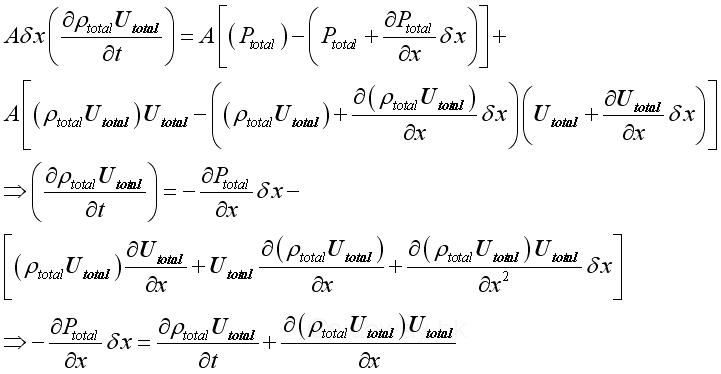Since both Utotal , ρtotal are a function of time, imply: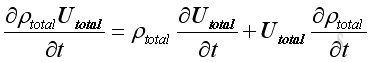Therefore, because of conservation of mass, the equation is: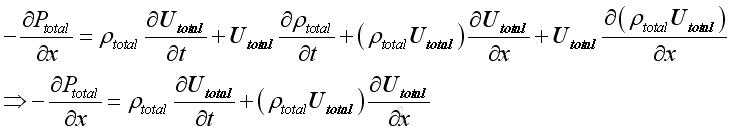As the medium fluid is assumed to be inviscid, the assumption of inviscid flow is valid for sound propagation and the euler's equation of motion can be applied.

The additional relationship between pressure and velocity is defined

## Energy Equation, 1D

For a control volume, from principle of energy conservation, rate of change of energy equal to rate of heat added and the net rate of energy flow into or out of the control volume minus the rate of work done. By neglecting heat energy and external work, and potential energy then: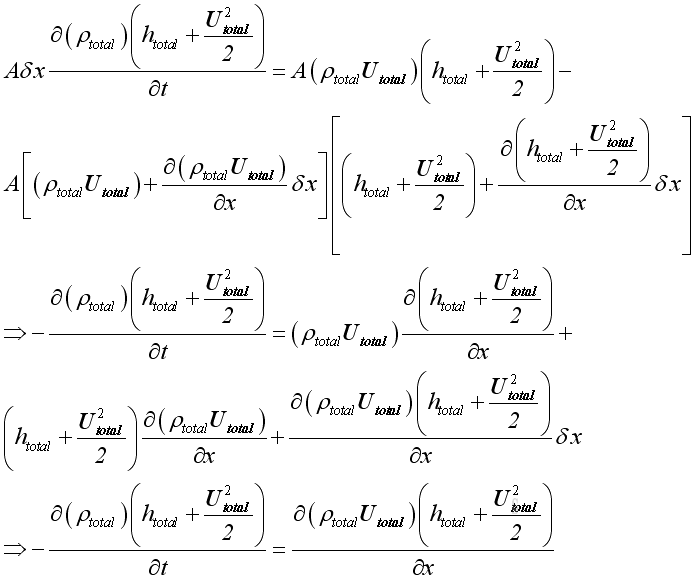The additional relationship between Enthalpy and velocity is defined.

©sidewayReferences

1. Michael P. Norton; Denis G. Karczub,, 2003, Fundamentals of Noise and Vibration Analysis for Engieer, Cambridge, United Kingdom
2. G. Porges, 1977, Applied Acoustics, Edward Arnold Limited, Britain
3. Douglas D. Reynolds, 1981, Engineering Principles of Acoustics:; Noise and Vibration Control, Allyn and Bacon, USA
4. Conrad J. Hemond, 1983, Engineering Acoustics & Noise Control, Prentice-Hall, USA
5. F. Fahy, 2001, Foundations of Engineering Acoustics, Academic Press, UK
6. D.A. Biew; C.H. Hansen, 1996, Engineering Noise Control: Theory and Practice, E & FN Spon, New YorkID: 100900019 Last Updated: 9/17/2010 Revision: 1 Ref:Home (5)

Business

Management

HBR (3)

Information

Recreation

Hobbies (7)

Culture

Chinese (1097)

English (336)

Reference (66)

Computer

Hardware (149)

Software

Application (187)

Digitization (24)

Numeric (19)

Programming

Web (644)CSS (SC)

ASP.NET (SC)

HTML

Knowledge Base

Common Color (SC)

Html 401 Special (SC)

OS (389)MS Windows

Windows10 (SC)

.NET Framework (SC)

DeskTop (7)

Knowledge

Mathematics

Formulas (8)

Number Theory (206)

Algebra (20)

Trigonometry (18)

Geometry (18)

Calculus (67)

Complex Analysis (21)

Engineering

Tables (8)

Mechanical

Mechanics (1)

Rigid Bodies

Statics (92)

Dynamics (37)

Fluid (5)

Control

Acoustics (19)

Biology (1)

Geography (1)

Latest Updated Links

Copyright © 2000-2019 Sideway . All rights reserved Disclaimers last modified on 10 Feb 2019